Study P1 Mathematics Subtraction within 20 - Geniebook# Subtraction Within 20

## Subtraction Within 10

Example:

James had some paper clips. He lost some of them. How many paper clips did James have left?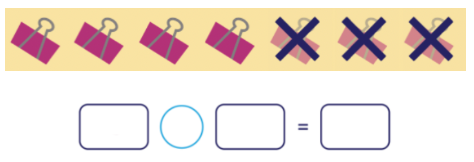He had __________ paper clips left.

4

Explanation:

Count the total number of paper clips and subtract the number of paper clips that are crossed out. We get: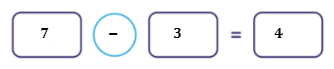Question 1:

Rachel had 8 bottles of water. She gave away 5 bottles of water. How many bottles of water does Rachel have left ?1. 1
2. 2
3. 3
4. 13

(3) 3

Explanation: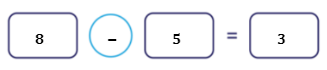Question 2:

There are 10 ducks. 2 ducks are in the pond. How many of them are not in the pond?(4) 8

Explanation: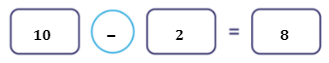Question 3:

Mr Tan has 10 pens. He gives away 3 pens. How many pens does he have left?1. 6
2. 7
3. 8
4. 13

(2) 7

Explanation: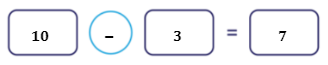## Subtraction With 20 By Subtracting The Ones First

Example:

Nina had 17 pencils. She lost 6 pencils. How many pencils did she have left?Nina has __________ pencils left.

11

Explanation:Split 17 into 10 and 7. Subtract 6 from 7. Add the answer to 10.

Step 1:

$$17 = 10 + 7$$

Step 2:

Subtract the ones.

Step 3:

\begin{align}​ 7 – 6 &= 1 \\ 10 + 1 &= 11 \end{align}

Question 1:

Complete the equation.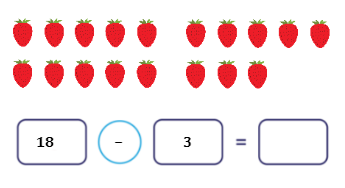1. 13
2. 14
3. 15
4. 16

(3) 15

Explanation:

Split 18 into 10 and 8. Subtract 3 from 8. Add the answer to 10.

Step 1:

$$18 = 10 + 8$$

Step 2:

Subtract the ones.

Step 3:

\begin{align}​ 8 – 3 &= 5 \\ 10 + 5 &= 15 \end{align}

Question 2:

Complete the equation.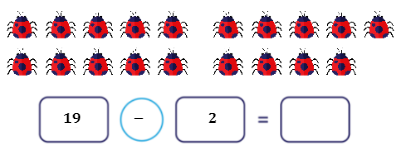1. 13
2. 15
3. 16
4. 17

(4) 17

Explanation:

Split 19 into 10 and 9. Subtract 2 from 9. Add the answer to 10.

Step 1:

$$19 = 10 + 9$$

Step 2:

Subtract the ones.

Step 3:

\begin{align}​ 9 – 2 &= 7 \\ 10 + 7 &= 17 \end{align}

## Subtraction With 20 By Subtracting From 10 First

Example:

Amy had 16 buttons. She gave 8 buttons to her sister. How many buttons did Amy have left?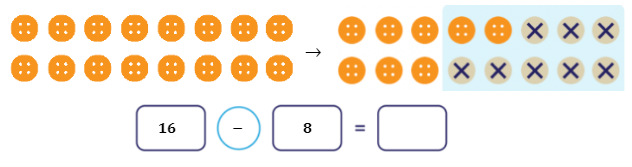8

Explanation:

Split 16 into 10 and 6. Subtract 8 from 10. Add the answer to 6.

Step 1:

$$16 = 6 + 10$$

Step 2:

Subtract from 10 first.

Step 3:

\begin{align}​ 10 - 8 &= 2 \\ 6 + 2 &= 8 \end{align}

Question 1:

Fill in the blank with the correct answer.$$13 - 5 =$$ __________

1. 8
2. 9
3. 12
4. 18

(1) 8

Explanation:

Step 1:

$$13 = 10 + 3$$

Step 2:

Subtract from 10.

Step 3:

\begin{align}​ 10 - 5 &= 5 \\ 5 + 3 &= 8 \end{align}

Question 2:

Fill in the blank with the correct answer:$$20 - 2 =$$ __________

1. 8
2. 10
3. 12
4. 18

(4) 18

Explanation:

Step 1:

$$20 = 10 + 10$$

Step 2:

Subtract from 10.

Step 3:

\begin{align}​ 10 - 2 &= 8 \\ 8 + 10 &= 18 \end{align}

Question 3:

Fill in the blank:

$$17 - 9 =$$ __________

1. 7
2. 8
3. 10

(2) 8

Explanation:

Step 1:

$$17 = 10 + 7$$

Step 2:

Subtract from 10.

Step 3:

\begin{align}​ 10 - 9 &= 1 \\ 1 + 7 &= 8 \end{align}

Question 4:

Which of the following subtraction equations best represents the diagram below?1. $$19 - 6 = 12$$
2. $$19 - 6 = 13$$
3. $$20 - 6 = 13$$
4. $$20 - 6 = 14$$

(2) $$19 - 6 = 13$$

Explanation:

Identify the total number of cupcakes and subtract the 6 cupcakes that are crossed out.

Step 1:

$$19 = 10 + 9$$

Step 2:

Subtract from 10.

Step 3:

\begin{align}​ 10 - 6 &= 4 \\ 4 + 9 &= 13 \end{align}

Question 5:

Which of the following equations best represents the diagram below?1. $$13 + 2 = 5$$
2. $$13 - 2 = 11$$
3. $$13 - 3 = 10$$
4. $$13 - 10 = 3$$

(2) $$13 - 2 = 11$$

Explanation:

Identify the total number of pumpkins and subtract the 2 pumpkins that are crossed out.

Step 1:

$$13 = 10 + 3$$

Step 2:

Subtract from 10.

Step 3:

\begin{align}​ 10 - 2 &= 8 \\ 8 + 3 &= 11 \end{align}

Question 6:

Which of the following equations best represents the diagram below?1. $$10 - 4 = 6$$
2.   $$4 + 6 = 10$$
3. $$12 - 4 = 8$$
4.   $$6 + 6 = 12$$

(3) $$12 - 4 = 8$$

Explanation:

Identify the total number of birds and subtract the 4 birds that are crossed out.

Step 1:

$$12 = 10 + 2$$

Step 2:

Subtract from 10 first.

Step 3:

\begin{align}​ 10 - 4 &= 6 \\ 6 + 2 &= 8 \end{align}

Question 7:

There were 17 apples. Tom ate 3 of them. How many apples are left now?1. $$14 - 3 = 11$$
2. $$14 - 4 = 10$$
3. $$17 - 4 = 13$$
4. $$17 - 3 = 14$$

(4) $$17 - 3 = 14$$

Explanation:

Identify the total number of apples and subtract the 3 apples that were eaten.

Step 1:

$$17 = 10 + 7$$

Step 2:

Subtract from 10 first.

Step 3:

\begin{align}​ 10 - 3 &= 7 \\ 7 + 7 &= 14 \end{align}

Question 8:

Which of the following is equal to 7?(4) $$15 - 8 = 7$$

Explanation:

1. $$20 - 3 = 17$$
2. $$19 - 7 = 12$$
3. $$18 - 3 = 15$$
4. $$15 - 8 = 7$$

Question 9:

Which of the following is not equal to 11?(2) $$16 - 6 = 10$$

Explanation:

1. $$10 + 1 = 11$$
2. $$16 - 6 = 10$$
3. $$13 - 2 = 11$$
4.   $$9 + 2 = 11$$

Question 10:

Which of the following number sentences is correct?(2) $$13 - 8 = 5$$

Explanation:

1. $$10 - 7 = 3 \quad \text{ (wrong)}$$
2. $$13 - 8 = 5 \quad\text{ (correct)}$$
3. $$19 - 6 = 13 \quad\text{(wrong)}$$
4. $$12 - 8 = 4 \quad\text{ (correct)}$$

Question 11:

Which of the following number sentences has the greatest answer?(2) $$16 - 3 = 13$$

Explanation:

1. $$14 - 7 = 7$$
2. $$16 - 3 = 13$$
3. $$17 - 8 = 9$$
4. $$20 - 9 = 11$$

Out of all the answers, 13 is the greatest.

• Subtraction Within 20

Continue Learning
Numbers To 10 Picture Graphs 1
Shapes 1 Ordinal Numbers
Subtraction Within 20 Addition Within 10
Addition And Subtraction Within 10 Numbers To 20
Addition Within 20 Numbers To 100PrimaryPrimary 1EnglishMaths
Numbers To 10
Picture Graphs 1
Shapes 1
Ordinal Numbers
Subtraction Within 20
Numbers To 20
Numbers To 100Primary 2Primary 3Primary 4Primary 5Primary 6SecondarySecondary 1Secondary 2Secondary 3Secondary 4